🐳 我就是我🐳
2021-11-28 09:27:57 2021-11-28
48
0

论文标题：DEEP GRAPH INFOMAX 　　论文方向：图像领域 　　论文来源：2019 ICLR 　　论文链接：https://arxiv.org/abs/1809.10341 　　论文代码：https://github.com/PetarV-/DGI

摘要

1.  DGI，一种以

1.

DGI 依赖于最大限度地扩大图增强表示和目前提取到的图信息之间的互信息 。

1.  与大多数以前使用 GCN 进行无监督学习的方法相比，

DGI不依赖于随机游走目标 ，并且很容易适用于直推式学习和归纳式学习。

1 介绍

DMI 训练一个编码模型来最大化高阶全局表示和输入的局部部分的互信息 。这鼓励编码器携带出现在所有位置的信息类型（因此是全局相关的），例如类标签的情况。

3 DGI Methodology

3.1 基于图的无监督学习

$\varepsilon: \mathbb{R}^{N \times F} \times \mathbb{R}^{N \times N} \rightarrow \mathbb{R}^{N \times F^{\prime}}$ ，可以形式化的表示为

$\mathcal{E}(\boldsymbol{X}, \boldsymbol{A})=\boldsymbol{H}=\left{\overrightarrow{h_{1}}, \overrightarrow{h_{2}}, \ldots, \overrightarrow{h_{N}}\right}$ ，其中 $H$ 代表高阶表示, 并且每个节点 $i$ 满足 $\overrightarrow{h_{i}} \in \mathbb{R}^{F^{\prime}}$ 。所得到的节点特征的高阶表示可以用于各种下游任务，例如节点分类任务。 在这里，我们将

3.2 局部-全局互信息最大化

DGI 的核心思想在于通过最大化局部互信息来训练编码器——即 DGI 寻求获得节点（即局部）表示，以捕获整个图的全局信息（表示为summary vector，$\vec{s}$）。

$\mathcal{R}: \mathbb{R}^{N \times F} \rightarrow \mathbb{R}^{F}$ ，利用它将获得的 patch representations 总结为图级别的表示。上述过程可以总结为

$\vec{s}=\mathcal{R}(\mathcal{E}(\boldsymbol{X}, \boldsymbol{A}))$ 。

$\mathcal{D}: \mathbb{R}^{F} \times \mathbb{R}^{F} \rightarrow \mathbb{R}$ ， 这样 $\mathcal{D}\left(\vec{h}_{i}, \vec{s}\right)$ 表示分配给这个 patch-summary 对的概率分数（对于包含在 summary 中的 patch 应该更高) 。

$\mathcal{D}$ 的负样本由 $(\boldsymbol{X}, \boldsymbol{A})$ 的 summary vector $\vec{s}$ 与一个可选择的图 $(\widetilde{\boldsymbol{X}}, \widetilde{\boldsymbol{A}})$  的 patch representations $\vec{h}_{j}$ 提供。在多图的数据集中，$(\widetilde{\boldsymbol{X}}, \widetilde{\boldsymbol{A}})$  可以通过训练集的其他元素获得。但是，对于单个图，需要一个显式（随机 ) corruption function，

$\mathcal{C}: \mathbb{R}^{N \times F} \times \mathbb{R}^{N \times N} \rightarrow \mathbb{R}^{M \times F} \times \mathbb{R}^{M \times M}$  来生成负样本的图  $(\widetilde{\boldsymbol{X}}$，$\widetilde{\boldsymbol{A}})$ 。 上述过程可以表述为

$(\widetilde{\boldsymbol{X}}, \widetilde{\boldsymbol{A}})=\mathcal{C}(\boldsymbol{X}, \boldsymbol{A})$

$\mathcal{L}=\frac{1}{N+M}\left(\sum \limits {i=1}^{N} \mathbb{E}{(\mathbf{X}, \mathbf{A})}\left[\log \mathcal{D}\left(\vec{h}{i}, \vec{s}\right)\right]+\sum \limits{j=1}^{M} \mathbb{E}{(\tilde{\mathbf{X}}, \tilde{\mathbf{A}})}\left[\log \left(1-\mathcal{D}\left(\overrightarrow{\widetilde{h}}{j}, \vec{s}\right)\right)\right]\right)$

3.3 DGI概述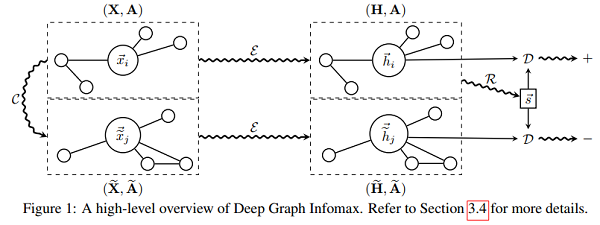1. 通过 corruption function 得到负样本实例: $(\widetilde{X}, \widetilde{\boldsymbol{A}}) \sim \mathcal{C}(\boldsymbol{X}, \boldsymbol{A})$ 。
2. 通过编码器获得输入图的 patch representations $\overrightarrow{h_{i}}: \boldsymbol{H}=\mathcal{E}(\boldsymbol{X}, \boldsymbol{A})=\left{\overrightarrow{h_{1}}, \overrightarrow{h_{2}}, \ldots, \overrightarrow{h_{N}}\right}$
3. 通过编码器获得负样本的 patch representations $\vec{h}{j}: \widetilde{H}=\mathcal{E}(\widetilde{X}, \widetilde{A})=\left{\vec{h}{1}, \vec{h}{2}, \ldots, \widetilde{h}{M}\right}$
4. 通过 Readout 函数传递输入图的 patch representations 来得到图级别的 summary vector: $\vec{s}=\mathcal{R}(\boldsymbol{H})$ 。
5. 通过梯度下降法最小化目标函数式 (1)，更新参数 $\mathcal{E}, \mathcal{R}, \mathcal{D}$。

4 实验

4.1 数据集

1.  在 Cora、Citeseer 和 Pubmed 引文网络上对研究论文进行主题分类。
2.  以Reddit帖子为模型预测社交网络的社区结构。
3.  对蛋白质-蛋白质相互作用（PPI）网络中的蛋白质作用进行分类，需要对未见网络进行归纳。

4.2 实验设置

4.2.1 直推式学习

$\mathcal{E}(\mathbf{X}, \mathbf{A})=\sigma\left(\hat{\mathbf{D}}^{-\frac{1}{2}} \hat{\mathbf{A}} \hat{\mathbf{D}}^{-\frac{1}{2}} \mathbf{X} \boldsymbol{\Theta}\right)$

PReLU （parametric ReLU）。$\Theta \in R^{F \times F^{\prime}}$ 是应用于每个节点的可学习线性变换。

4.2.2 大图上的归纳式学习

$\operatorname{MP}(\mathbf{X}, \mathbf{A})=\hat{\mathbf{D}}^{-1} \hat{\mathbf{A}} \mathbf{X} \Theta$

$\widehat{D} ^{-1}$ 实际上执行的是标准化的和（因此是 mean-pooling）。尽管上式明确指定了邻接矩阵和度矩阵，但并不需要它们：因为 Const-GAT 模型中使用的持续关注机制可以观察到相同的归纳行为。

对于 Reddit 数据库，DGI 的编码器是一个带有跳跃连接的三层均值池模型：

$\widetilde{\mathrm{MP}}(\mathbf{X}, \mathbf{A})=\sigma\left(\mathbf{X} \Theta^{\prime} | \operatorname{MP}(\mathbf{X}, \mathbf{A})\right) \quad \mathcal{E}(\mathbf{X}, \mathbf{A})=\widetilde{\mathrm{MP}}{3}\left(\widetilde{\mathrm{MP}}{2}\left(\widetilde{\mathrm{MP}}_{1}(\mathbf{X}, \mathbf{A}), \mathbf{A}\right), \mathbf{A}\right)$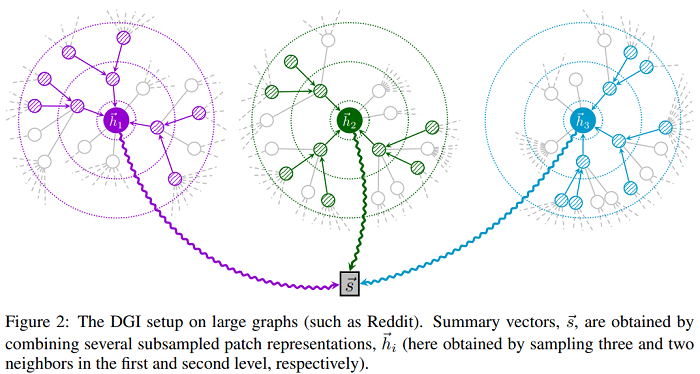4.2.3 多图上的归纳式学习

$\mathbf{H}{1}=\sigma\left(\operatorname{MP}{1}(\mathbf{X}, \mathbf{A})\right)$

$\mathbf{H}{2}=\sigma\left(\mathbf{M P}{2}\left(\mathbf{H}{1}+\mathbf{X} \mathbf{W}{\text {skip }}, \mathbf{A}\right)\right)$

$\mathcal{E}(\mathbf{X}, \mathbf{A})=\sigma\left(\mathbf{M P}{3}\left(\mathbf{H}{2}+\mathbf{H}{1}+\mathbf{X} \mathbf{W}{\text {skip }}, \mathbf{A}\right)\right)$

其中，$W_{skip}$ 是一个可学习的投影矩阵。

$\mathcal{R}(\mathbf{H})=\sigma\left(\frac{1}{N} \sum \limits {i=1}^{N} \vec{h}{i}\right)$

作者通过应用一个简单的双线性评分函数对图级别的 summarize-patch representation 对进行评分：

$\mathcal{D}\left(\vec{h}{i}, \vec{s}\right)=\sigma\left(\vec{h}{i}^{T} \mathbf{W} \vec{s}\right)$

4.3 结果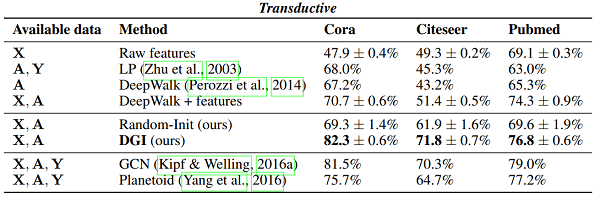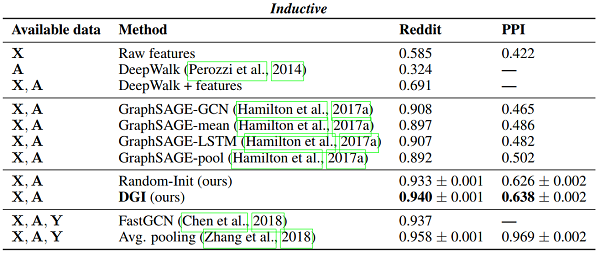GCN" 对应于以监督方式训练的两层 DGI 编码器。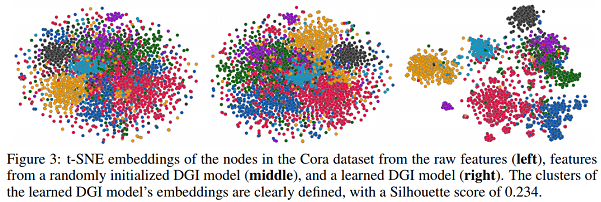5 参考

1 Deep Graph Infomax